# Modeling a Foucault Pendulum

This example shows how to model a Foucault pendulum. The Foucault pendulum was the brainchild of the French physicist Leon Foucault. It was intended to prove that Earth rotates around its axis. The oscillation plane of a Foucault pendulum rotates throughout the day as a result of axial rotation of the Earth. The plane of oscillation completes a whole circle in a time interval T, which depends on the geographical latitude.

Foucault's most famous pendulum was installed inside the Paris Pantheon. This was a 28kg metallic sphere attached to a 67 meter long wire. This example simulates a 67 meter long pendulum at the geographic latitude of Paris.

The simplest way to solve the Foucault pendulum problem in Simulink® is to build a model that solves the coupled differential equations for the system. This model is shown in Figure 1. The equations that describe the Foucault pendulum are given below. For details on the physics of the model and the derivation of these equations, see Analysis and Physics.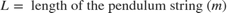### Opening the Model

Type sldemo_foucault in MATLAB® Command Window to open this model. This model logs simulation data to the variable sldemo_foucault_output. Logged signals have a blue indicator. For more information, see Configure a Signal for Logging.Figure 1: The Foucault pendulum model

### Initial Conditions

This model loads the constants and initial conditions from the sldemo_foucault_data.m file. The contents of this file are shown in Table 1 below. You can modify simulation parameters directly in MATLAB workspace. The initial amplitude of the pendulum must be small compared to pendulum length, because the differential equations are valid only for small oscillations.

Table 1: Initial conditions

g = 9.83; % acceleration of gravity (m/sec^2) L = 67; % pendulum length (m) initial_x = L/100; % initial x coordinate (m) initial_y = 0; % initial y coordinate (m) initial_xdot = 0; % initial x velocity (m/sec) initial_ydot = 0; % initial y velocity (m/sec) Omega=2*pi/86400; % Earth's angular velocity of rotation about its axis (rad/sec) lambda=49/180*pi; % latitude in (rad)

### Running the Simulation

Press the "Play" button on the toolbar in the model window to run the simulation. The simulation will use a variable step stiff solver, ode23t. It will simulate a Foucault pendulum for 3600 seconds (you can change the simulation time). The model uses a default relative tolerance RelTol = 1e-6.Figure 2: Foucault pendulum simulation results (simulation time of 3600 sec)

### Results

The simulation results are shown in Figure 2 above. The simulation calculates the pendulum x and y coordinates, and the x and y velocity components of the pendulum.

The pendulum oscillation plane completes a 360 degree sweep in more than 24 hours. The sweep period is a function of geographic latitude lambda (see derivation in Analysis and Physics ).Figure 3: The Animation block shows how much the pendulum oscillation plane rotates in an hour

After you run the simulation, double click the animation block to animate the results.

• Note: The "Animate Results" portion of the example requires Signal Processing Toolbox™. Double-clicking on the animation block will cause an error if it is not installed. All other parts of the example will function correctly without the Signal Processing Toolbox.

The sldemo_foucault_animate.m file plots the position of the pendulum bob at different points in time. You can clearly see how the pendulum oscillation plane rotates.

• Note: If you are running the simulation at a large relative tolerance, the result will be numerically unstable over a long period of time. Make sure that you are using a stiff variable-step solver. Read more about numerical instability of stiff problems and solver performance in the "Exploring Variable-Step Solvers Using a Stiff Model" example.

### Closing the Model

Close the model. Clear generated data.

### Analysis and Physics

This section analyzes the Foucault pendulum and describes the physics behind it. The pendulum can be modeled as a point mass suspended on a wire of length L. The pendulum is located at the geographical latitude lambda. It is convenient to use the reference frames shown in Figure 4: the inertial frame I (relative to the center of the Earth), and the non-inertial frame N (relative to an observer on Earth's surface). The non-inertial frame accelerates as a result of rotation.Figure 4: The Inertial and Non-Inertial Frames for the Problem

Point O is the origin of the non-inertial frame N. It is the point on the surface of the earth beneath the point of suspension of the pendulum. The non inertial frame is chosen such that the z-axis points away from the center of the Earth and is perpendicular to Earth's surface. The x-axis points south and the y-axis points west.

As mentioned in the introduction, the oscillation plane of a Foucault pendulum rotates. The oscillation plane completes a full rotation in time Trot given by the following formula, where Tday is the duration of one day (i.e. the time it takes the Earth to revolve around its axis once).The sine factor requires further discussion. It is often incorrectly assumed that the oscillation plane of the pendulum is fixed in the inertial frame relative to the center of the Earth. This is only true at the north and south poles. To eliminate this confusion, think about the point S (see Figure 4), where the pendulum is suspended. In the inertial frame I, point S moves on a circle. The pendulum bob is suspended on a wire of constant length. For simplicity ignore the air friction. In the inertial frame I, there are only two forces that act on the bob - the wire tension T and the gravitational force Fg.

The vector r gives the position of the pendulum bob, B (see Figure 4). Newton's second law states that the sum of all forces acting on a body equals the mass times the acceleration of the body.Throughout this proof, the dots denote time derivatives, arrows denote vectors, caps denote unitary vectors (i, j, and k along x, y, and z axes). A dot above the vector arrow indicated the time derivative of the vector. An arrow above the dot indicated the vector of the time derivative. See the difference between total acceleration and radial acceleration below.

Total Acceleration:The acceleration of gravity points towards the center of the earth (negative z-direction).Decompose the acceleration term: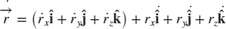The time derivatives of unit vectors appear because the non-inertial reference frame N is rotating in space. This means that the unitary vectors i, j, and k rotate in space. Their time derivatives are given below. Omega is Earth's angular velocity of revolution around its axis. The scalar Omega is the value of the angular velocity. The vectorial Omega is the vector angular velocity. Its direction is determined by the right hand rule.Rewrite the time derivative of the vector r relative to Omega.Similarly, express the second time derivative of the vector r.To simplify this equation, assume that Omega for Earth is very small. This allows us to ignore the third term in the equation above. In fact, the second term (which is already much smaller than the first term) is four orders of magnitude greater than the third term. This reduces the equation to the following form:Newton's Second Law can be written and decomposed into x,y, and z components as follows: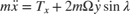The angular amplitude of oscillations is small. Therefore, we can ignore the vertical velocity and vertical acceleration (z-dot and z-double-dot). The string tension components can be expressed using small angle approximations, which also considerably simplify the problem, making it two-dimensional (see below).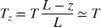### Characteristic Differential Equations

Finally the physics of the problem can be described by the system of coupled equations given below. The x and y coordinates specify the position of the pendulum bob as seen by an observer on Earth.### Analytic Solution (Approximate)

The following is an analytic solution to the Foucault pendulum problem. Unfortunately, it is not exact. If you try to substitute the analytic solution into the differential equations, uncanceled terms of the order Omega squared will remain. However, because Omega is very small, we can ignore the uncanceled terms for practical purposes.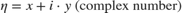### Actual Differential Equation System Is Asymmetric

During the derivation, terms involving Omega squared were ignored. This resulted in xy symmetry in the differential equations. If the Omega squared terms are taken into consideration, the differential equation system becomes asymmetric (see below).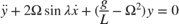You can easily modify the current Foucault pendulum model to account for asymmetric differential equations. Simply edit the corresponding Gain blocks that contain g/L and add the necessary expression. This change will introduce a very small overall correction to the numerical result.